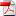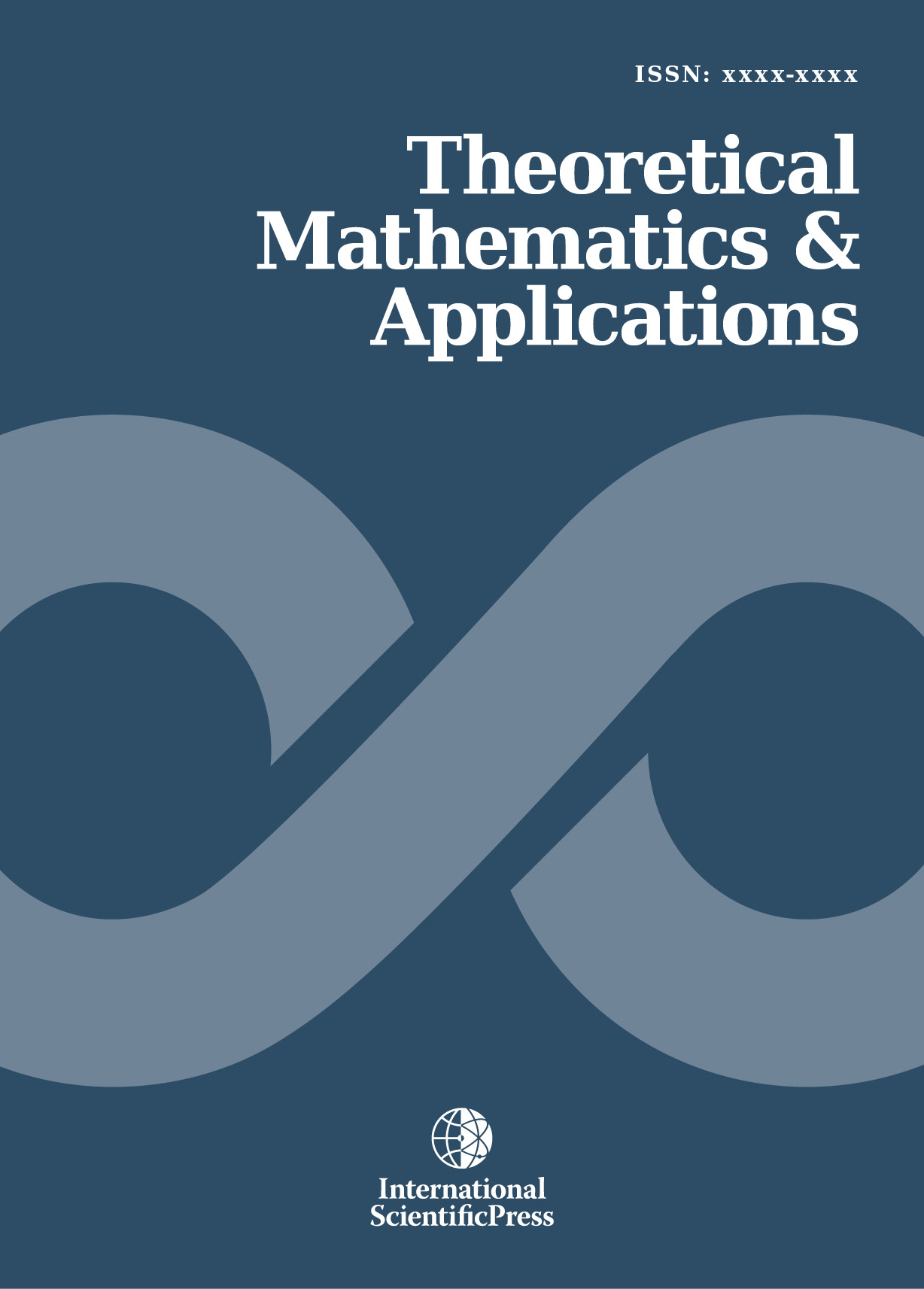# Theoretical Mathematics & Applications

#### Discreteness of the solutions to equations of mathematical physics

•[ Download ]
• Times downloaded: 1687
• Abstract

The equations of mathematical physics, which describe actual processes, are defined on manifolds (tangent or others) that are not integrable. The solutions of equations on such manifolds cannot be functions since the derivatives of such solutions do not made up a differential. The exact solutions (functions), which are possible only on integrable structures, can be realized only discretely under the realization of additional conditions. The process of realization of discrete solutions reveals the mechanism of generation of integrable structures, which format integrable manifolds, and emergence of physical structures, which made up physical fields and an occurrence of waves, eddies and so on.ISSN: 1792-9687 (Print)
1792-9709 (Online)